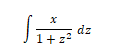## Integration in Python using Sympy

In this section, we discuss how to find integration in Python Sympy. Sympy is a Symbolic Python library in Python defined through symbols function. We also discuss Integral function in Mathematics and an integral module in Sympy. Then we moved to how to calculate integration in Python using Sympy with Python code.

## Integral function in Mathematics

Mathematics functions as an integration function. lt describes displacement, area, volume, and other concepts that arise from a mixture of infinite data. In calculus the Integration is one of the main operations from two, with its inverse operation, differentiation, being the other. Given a function f of a real variable x and an interval [a, b] of the real line, the fixed integral.Informally defined in the x-plane as the signed region of the region bounded by the graph of f, the x-axis and the vertical lines x = a and x = b. The area above the x-axis joins the total and decreases from the total below the x-axis.

The operation of integration, up to an additive constant, is the inverse of the operation of discrimination. For this reason, the term integral can also refer to the related notion of the additive, a function f whose derivative is the given function f.

## Integral module in Sympy

The integral module in Sympy implements methods for computing fixed and indefinite integrals of expressions.

The main method in this module is to integrate()

Integrate(f, x) returns indefinite integral fdx.

Integrate(f, (x, a, b)) gives definite integral.

Integration can be carried out by integrate command.

1. integrate(f)

2. integrate(f,x) where x is a variable

3. integrate(f,x, ll, ul) where ll - lower limit and ul - upper limit

Now let's check the way to implement integration using integrate function.

1. Find the integration of the following function using Sympy.from sympy import *
x = symbols('x')
f = 2*x
y = integrate(f)
print(y)


Output: x**2

2. Find the integration of the following function using Sympy.from sympy import *
x = symbols('x')
f = 2*x
y = integrate(f,x)
print(y)
Output: x**2



3. Find the integration of the following function using Sympy.from sympy import *
x = symbols('x')
f = sin(x)
y = integrate(f,x)
print(y)
Output: -cos(x)

4. Find the integration of the following function using Sympy.from sympy import *
x = symbols('x')
f = exp(x)
y = integrate(f,x)
print(y)
Output: exp(x)

5. Find the integration of the following function using Sympy.from sympy import *
x = symbols('x')
f = log(x)
y = integrate(f,x)
print(y)
Output: x*log(x) - x

6. Find the integration of the following function using Sympy.from sympy import *
x = symbols('x')
z = symbols('z')
f = x/1+z**2y = integrate(f,x)
print(y)
Output: x**2/2 + x*z**2

7. Find the integration of the following function using Sympy.from sympy import *
x = symbols('x')
f = x/1+x**2y = integrate(f,x)
print(y)
Output: x**3/3 + x**2/2

8. Find the integration of the following function using Sympy.from sympy import *
x = symbols('x')
f = x
y = integrate(f,(x,4,9))
print(y)

Output:65/2